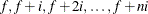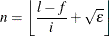### Range Expression

expression-1 .. expression-nBY expressionThe range expression returns the set of numbers from the specified arithmetic progression. The sequence proceeds from the left operand value up to the right operand limit. The increment between numbers is 1 unless a different value is specified with a BY clause. If the increment is negative, then the progression is from the left operand down to the right operand limit. The result can be an empty set.

For compatibility with the DATA step iterative DO loop construct, the keyword TO can substitute for the range (..) operator.

The limit value is not included in the resulting set unless it belongs in the arithmetic progression. For example, the following range expression does not include 30:

proc optmodel;
put (10..30 by 7); /* outputs {10,17,24} */


The actual numbers that the range expression f..l by i produces are in the arithmetic sequencewhereandrepresents the relative machine precision. The limit is adjusted to avoid arithmetic roundoff errors.

PROC OPTMODEL represents the set specified by a range expression compactly when the value is stored in a parameter location, used as a set operand of an IN or NOT IN expression, used by an iterative DO loop, or used in an index set. For example, the following expression is evaluated efficiently:

   999998.5 IN 1..1000000000